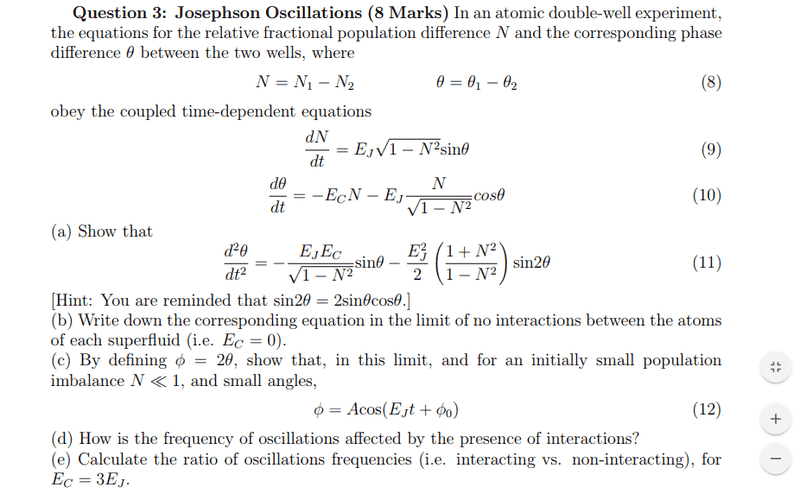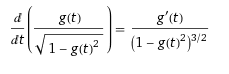# Josephson Oscillations

CMJ96

## Homework Statement[/B]

## Homework Equations

Sorry about this, I had to put this into wolfram alpha as for some reason it would not work in latex## The Attempt at a Solution

Assuming Ec and Ej are not dependant on time, I have differentiated the first term in equation 10 with respect to time, and replaced the relevant terms with equation 9. The second term I have differentiated the fraction containing N's using the expression in the "relevant equations" section . This has resulted in the following expression

$$\frac{d^2 \theta}{dt^2} = -E_c E_J \left(1-N^2 \right)^{\frac{1}{2}} sin(\theta) - \frac{E_{J}^2 sin(\theta) cos(\theta)}{1-N^2}$$
Can someone help me figure out why I am missing a factor of ##1/(1-N^2) ## in the first term and a factor of ##1+N^2## in the second term? My equation looks similar but I appear to have missed a step

## Answers and Replies

Mentor
It appears you forgot the derivative of the ##\cos \theta## term when you took the derivative of eq. (10).

CMJ96
I see, would I have to apply the product rule to the second term in order to differentiate the ##cos \theta ## properly?

$$\frac{d^2 \theta}{dt^2}= -E_c E_J (1-N^2)^{\frac{1}{2} } sin \theta - \frac{E_J ^2 sin \theta cos \theta }{1-N^2} + \frac{E_J N sin \theta } {(1-N^2)^{\frac{1}{2}} }$$

Mentor
I see, would I have to apply the product rule to the second term in order to differentiate the ##cos \theta ## properly?
Yes, properly, meaning
$$\frac{d}{dt} \cos \theta = -\sin \theta \frac{d \theta}{dt}$$

CMJ96
Yes, properly, meaning
$$\frac{d}{dt} \cos \theta = -\sin \theta \frac{d \theta}{dt}$$
Ahhhh yes, silly mistake..... now I'm getting the correct second term (I just have to use the trig identity to tidy it up) , the only issue is that the first term is slightly wrong, I can't see how I can get the ## (1-N^2)^{\frac{1}{2}} ## as a denominator

$$\frac{d^2 \theta}{dt^2} = -E_c E_J (1-N^2)^{\frac{1}{2}} sin \theta - \frac{E_J E_c N^2 sin \theta}{(1-N^2)^{1/2}} - \frac{-E_J ^2 (1+N^2) sin \theta cos \theta}{1-N^2}$$

Last edited by a moderator:
Mentor
Ahhhh yes, silly mistake..... now I'm getting the correct second term (I just have to use the trig identity to tidy it up) , the only issue is that the first term is slightly wrong, I can't see how I can get the ## (1-N^2)^{\frac{1}{2}} ## as a denominator
Then you seem to have still forgotten a term. When calculating
$$\frac{d}{dt} \cos \theta = -\sin \theta \frac{d \theta}{dt}$$
the ##\frac{d \theta}{dt}## should bring a term in ##E_C##, which eventually will modify the term in ##E_C E_J## in your equation for ##\frac{d^2 \theta}{dt^2}##.

CMJ96
Then you seem to have still forgotten a term. When calculating
$$\frac{d}{dt} \cos \theta = -\sin \theta \frac{d \theta}{dt}$$
the ##\frac{d \theta}{dt}## should bring a term in ##E_C##, which eventually will modify the term in ##E_C E_J## in your equation for ##\frac{d^2 \theta}{dt^2}##.
Ah yes, sorry, I forgot to include the 4th term in my reply, I have added it now, the denominator should be ##(1-N^2)^{\frac{1}{2}} ## but for some reason latex won't display it

Mentor
Ah yes, sorry, I forgot to include the 4th term in my reply, I have added it now, the denominator should be ##(1-N^2)^{\frac{1}{2}} ## but for some reason latex won't display it
I fixed it for you.

You now simply have to combine the two ##E_C E_J## terms into one and you'll be done.

CMJ96
I fixed it for you.

You now simply have to combine the two ##E_C E_J## terms into one and you'll be done.
I've got it now, thank you very much for the assistance!